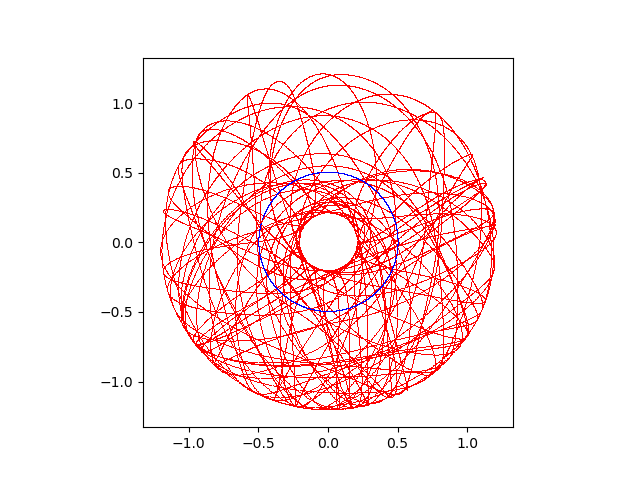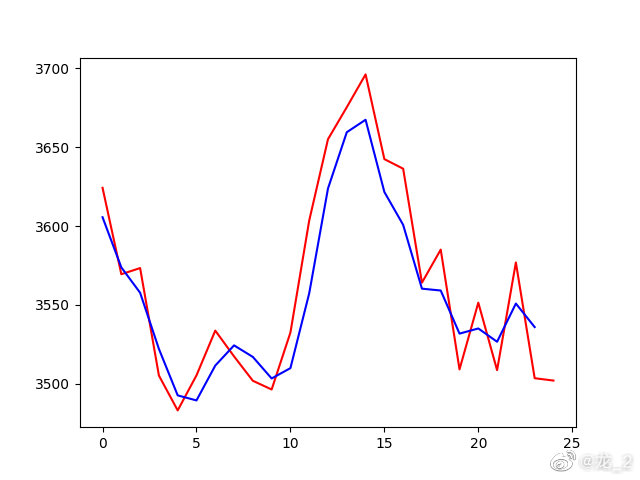# LongLong's Blog

## 使用Open Dynamics Engine实现刚体动力学仿真

#### 1. ODE的安装

ODE的安装非常简单，从其官方网站https://www.ode.org，找到在BitBucket中的代码库地址，使用git直接下载整个代码库，并使用cmake进行编译安装。此外也可以使用各Linux发行版的包管理器进行安装。

pacman -S ode
#下载源代码
git clone https://bitbucket.org/odedevs/ode.git
cmake .
make


python setup.py build
python setup.py install


#### 2. ODE的模块组成

ODE中对于整个模型分为世界World，刚体Body，约束（铰接）Joints ，力Force，几何体Geometry等。通过在World中增加Boby及Joints等连接约束关系，建立整个多刚体等力学模型，并通过不断循环时间步长通过积分来计算整个系统的下个状态。代码的基本框架如下

import ode;
#创建时间
world = ode.World();
#设置重力
world.setGravity((0, -9.8, 0));
#新建一个刚体
b1 = ode.Body(world);
#设置时间补偿
TIME_STEP = 0.01;
#进行1000次积分循环
for i in range(0, 1000):
#得到当前刚体的位置
x1, y1, z1 = b1.getPosition();
world.step(TIME_STEP);


#### 3. 双摆的运动模拟及双摆曲线的绘制

import numpy;
import ode;
from matplotlib import pyplot as plt;
import matplotlib.animation as animation;
if __name__ == '__main__':
#创建世界
world = ode.World();
world.setGravity((0, -9.8, 0));
#创建第一个质点
b1 = ode.Body(world);
#设置初始位置和速度
b1.setPosition((0, -0.5, 0))
b1.setLinearVel((10, 0, 0));
#设置质量和外形
m1 = ode.Mass();
m1.setSphereTotal(0.1, 0.05);
b1.setMass(m1);
#创建第二个质点
b2 = ode.Body(world);
#设置初始位置，默认初始速度为0
b2.setPosition((0.5, -1.0, 0));
#设置质量和外形
m2 = ode.Mass();
m2.setSphereTotal(0.1, 0.05);
b2.setMass(m2);
#创建第一个质点和环境的约束
j1 = ode.BallJoint(world);
j1.attach(b1, ode.environment);
j1.setAnchor((0.0, 0.0, 0.0));
#创建第二个质点和第一个质点之间的约束
j2 = ode.BallJoint(world);
j2.attach(b1, b2);
j2.setAnchor((0, -0.5, 0));
#设置时间步长
TIME_STEP = 0.01;
fig, ax = plt.subplots();
for i in range(0, 20000):
#分别获得两个质点的位置
x1, y1, z1 = b1.getPosition();
x2, y2, z2 = b2.getPosition();
#绘制当前的位置
plt.plot(x1, y1, 'b,');
plt.plot(x2, y2, 'r,');
#进入下一时刻
world.step(TIME_STEP);
#设置绘图的X和Y轴保持相同的比例
ax.set_aspect('equal');
#保持绘制的图片
plt.savefig('1.png');## 使用循环神经网络预测股价

#### 1. 获得训练数据

pip install pandas
pip install tensorflow==2.3.0


Tushare的使用非常简单，直接调用获取数据的方法即可返回数据集，需要传入的参数包括股票的代码，上证综合指数为sh000001，以及获取数据的日期区间。同时将数据按照时间索引进行排序，获取数据的代码如下

data = tushare.get_hist_data('sh000001', '2011-01-01', '2020-12-31');
data.index = data.index.astype('datetime64[ns]');
data = data.sort_index()['close'].values.reshape(-1, 1);


#### 2. 生成训练数据集

#归一化处理
scl = MinMaxScaler();
data = scl.fit_transform(data);
#预测使用的数据点个数及预测的数据点个数
look_back = 30;
forward_days = 1;
#生成训练数据集
x_train, y_train = [], [];
for i in range(0, len(data) - look_back - forward_days + 1):
x_train.append(data[i : (i + look_back)]);
y_train.append(data[(i + look_back) : (i + look_back + forward_days)]);
x_train = numpy.array(x_train);
y_train = numpy.array(y_train);


#### 3. 构造循环神经网络模型

#初始化循环神经网络模型
model = Sequential();
#第一层长短期记忆层
model.add(LSTM(100, input_shape=(look_back, 1), return_sequences=True));
#防止过拟合增加Dropout层
#第二层长短期记忆层
#最后的全连接层
#编译模型
#进行数据拟合
model.fit(x_train, y_train, epochs=100, batch_size=16);
#保存训练得到的模型
model.save(model_file);


import os;
os.environ['CUDA_VISIBLE_DEVICES'] = '-1';


#### 4. 进行预测

#获取预测需要的数据，并对数据进行处理
data = tushare.get_hist_data(no, '2021-01-01');
data.index = data.index.astype('datetime64[ns]');
data = data.sort_index()['close'].values.reshape(-1, 1);
data = scl.fit_transform(data);
#构造预测的输入数据集
x_test = [];
for i in range(0, len(data) - look_back - forward_days + 1):
x_test.append(data[i : (i + look_back)]);
x_test = numpy.array(x_test);
#使用模型对结果进行预测
y_test = model.predict(x_test);


import matplotlib.pyplot as plt;
plt.plot(scl.inverse_transform(y_test.reshape(-1, 1))[0:-1]);
plt.plot(scl.inverse_transform(data[look_back + 1:]));
plt.show();


#### 5.结果